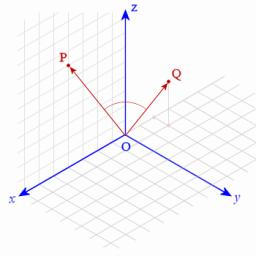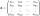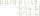# Cuboids

Two separate cuboids with different orientation in space. Determine the angle between them, knowing the direction cosine matrix for each separate cuboid. u1=(0.62955056, 0.094432584, 0.77119944) u2=(0.14484653, 0.9208101, 0.36211633)

Correct result:

a =  62.7801 °

#### Solution:We would be pleased if you find an error in the word problem, spelling mistakes, or inaccuracies and send it to us. Thank you!

Showing 1 comment:Matikar
use scalar products to determine angle between two 3D vectors (if direction cosines gives -> its unit vectors)Tips to related online calculators
For Basic calculations in analytic geometry is a helpful line slope calculator. From coordinates of two points in the plane it calculate slope, normal and parametric line equation(s), slope, directional angle, direction vector, the length of segment, intersections the coordinate axes etc.
Our vector sum calculator can add two vectors given by its magnitudes and by included angle.
Most natural application of trigonometry and trigonometric functions is a calculation of the triangles. Common and less common calculations of different types of triangles offers our triangle calculator. Word trigonometry comes from Greek and literally means triangle calculation.

## Next similar math problems:

• The determinantThe determinant of the unit matrix equals 7. Check how many rows the A matrix contains.
• Inverse matrixFind how many times is the larger determinant is the matrix A, which equals 9 as the determinant of its inverse matrix.
• The inverseThe inverse matrix for matrix A has a determinant value of 0.333. What value has a determinant of the matrix A?
• Inverse matrixFind out inverse by Gauss elimination or by reduction method. A=[2/3. 1 -3. 1/3]